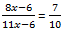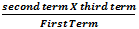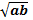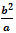# SBI PO Exam 2015: Quantitative Aptitude- Ratio and Proportion -Concept & Sample Questions

The banking team of jagranjosh.com has come up with concept and sample questions for Ratio and Proportion. The concept provided by us will help you to understand the topic. The sample questions offered by us are framed by keeping in view need of the question paper.

Created On: Apr 16, 2015 14:53 IST
Modified On: May 18, 2015 10:45 ISTThe banking team of jagranjosh.com has come up with concept and sample questions for Ratio and Proportion. The concept provided by us will help you to understand the topic. The sample questions offered by us are framed by keeping in view need of the question paper.Ratio

The ratio of two quantities in the same unit is a fraction that one quantity is of other. There cannot be ratio between quantities of different kinds.

Ratio of two quantities a and b are called terms of ratios.

The first term of the ratio is called antecedent, while the second term is known as consequent.

Thus, the ratio 5:9 represents 5/9 with antecedent 5 and consequent 9.1. Compound Ratio

When the numerators and denominators of two or more ratios are multiplies to obtain the new numerator and denominator, a new ratio is formed called the compounded ratio.

For example

The compound ratio of 2:3, 3:4, 5:6 is (2x3x5): (3x4x6) = 30:72 = 5:12

2. Duplicate Ratio

When a ratio is compounded with itself, and thus the resulting ratio is called the duplicate ratio of a given ratio.

Thus  a2 : b2 is the duplicate ratio of a:b

For Example

Two numbers are in the ratio 8:11. If 6 is subtracted from  given each, the resulting numbers are 7:10. Find the two numbers.

Solution

Let the given number be 8x and 11x

By hypothesis,80x-60x = 77x-42 or 3x = 18

x=6

Hence the numbers are 48 and 66

Proportion

Four quantities are said to be in proportion when the ratio of the first two quantities is same as the ratios of the last two quantities.

3:4 = 15:20, 3, 4, 15, 20 are in proportion

1. Continued Proportion:  Three quantities of the same kind are said to be in continued proportion, if a:b=b:c, then a, b, c are in continued Proportion.

2. Direct Proportion: In direct proportion, the ratio of the first two terms is equal to thew ratio of last two terms and said quantities are in proportion.

3. Indirect Proportion: In indirect proportion, a greater number require a smaller number and vice versa.

a. Fourth Proportional =b. Mean Proportion:c. Third Proportion =Comment ()

1 + 0 =
Post